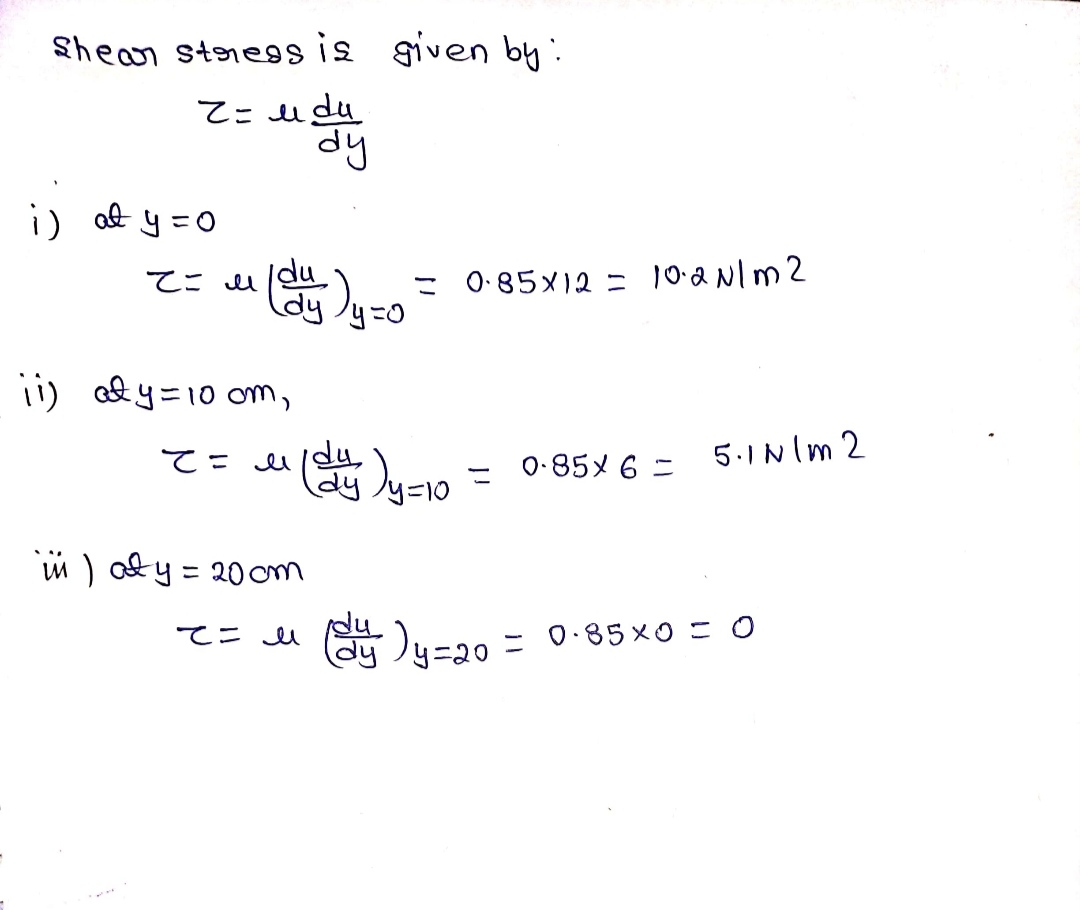# The velocity profile of a fluid over a plate is parabolic with the vertex 20 cm from the plate, where the velocity is 120 cm/sec. Calculate the velocity gradients and shear stresses at a distance of 0, 10, and 20 cm from the plate, if the viscosity of the fluid is 8.5 poise. u= 120 cm/sec 20 cm.

Question-AnswerCategory: Fluid MechanicsThe velocity profile of a fluid over a plate is parabolic with the vertex 20 cm from the plate, where the velocity is 120 cm/sec. Calculate the velocity gradients and shear stresses at a distance of 0, 10, and 20 cm from the plate, if the viscosity of the fluid is 8.5 poise. u= 120 cm/sec 20 cm.

The velocity profile of a fluid over a plate is parabolic with the vertex 20 cm from the plate, where the velocity is 120 cm/sec. Calculate the velocity gradients and shear stresses at a distance of 0, 10, and 20 cm from the plate, if the viscosity of the fluid is 8.5 poise. u= 120 cm/sec 20 cm.

Step 1

Velocity u=120cm/sec

Step 2Step 3